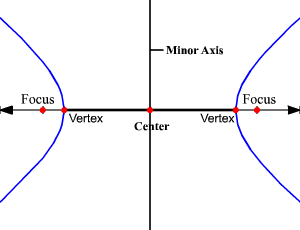# Major and Minor Axes of Conics

The axes of the conics are the axes of symmetry for the figure.  An axis of symmetry for a graph is a line in the plane of the conic that is a line of symmetry meaning that the two sides of the graph on either side of the axis look like mirror images of each other.

### Axes of Symmetry of a Circle:

A circle has infinitely many axes of symmetry because any line through the center of the circle divides the circle into symmetrical halves.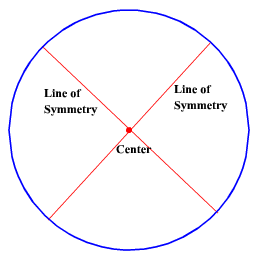### Axis of Symmetry of a Parabola:

The axis of symmetry of a parabola is the line passing through the focus and vertex of the parabola.  It is perpendicular to the directrix.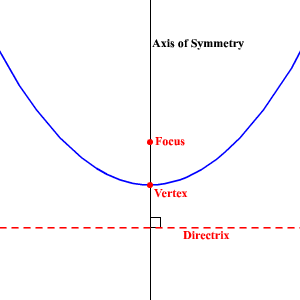### Major and Minor Axes of an Ellipse:

The major axis of an ellipse contains the longer of the two line segments about which the ellipse is symmetrical.  It is the line that passes through the foci, center and vertices of the ellipse.  It is considered the principle axis of symmetry.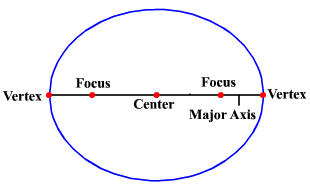The minor axis of an ellipse is the line that contains the shorter of the two line segments about which the ellipse is symmetrical.  It passes through the center of the ellipse and is perpendicular to the major axis.  It is an axis of symmetry.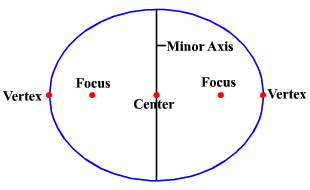### Major and Minor Axes of a Hyperbola:

The major axis of a hyperbola is the line that passes through the foci, center and vertices of the hyperbola.  It is considered the principle axis of symmetry.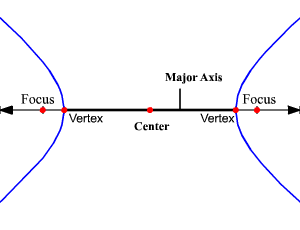The minor axis of a hyperbola is the line that passes through the center of the hyperbola and is perpendicular to the major axis.  It is also an axis of symmetry.